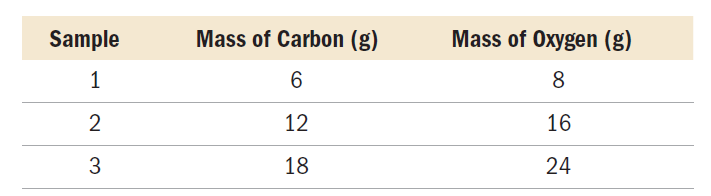×
Get Full Access to Chemistry: A Molecular Approach - 3 Edition - Chapter 1 - Problem 35e
Get Full Access to Chemistry: A Molecular Approach - 3 Edition - Chapter 1 - Problem 35e

×

# A chemist decomposes several samples of carbon monoxideISBN: 9780321809247 1

## Solution for problem 35E Chapter 1

Chemistry: A Molecular Approach | 3rd Edition

• Textbook Solutions
• 2901 Step-by-step solutions solved by professors and subject experts
• Get 24/7 help from StudySoup virtual teaching assistantsChemistry: A Molecular Approach | 3rd Edition

4 5 1 274 Reviews
22
2
Problem 35E

A chemist decomposes several samples of carbon monoxide into carbon and oxygen and weighs the resultant elements. The results are shown in the table.a. Do you notice a pattern in these results? Next, the chemist decomposes several samples of hydrogen peroxide into hydrogen and oxygen. The results are shown in the table:b. Do you notice a similarity between these results and those for carbon monoxide in part a?

c. Can you formulate a law from the observations in a and b?

d. Can you formulate a hypothesis that might explain your law in c?

Step-by-Step Solution:
Step 1 of 3

Solution 35E: There are two types of number in scientific work. (i) exact - exactly the values are known when it is measured. The values which are obtained from any measurement is completely certain value (ii) Inexact number- uncertainty in the numbers. The measured values are uncertain. (a) the mass of a 3 by 5-inch index card It is an inexact number. The mass of a 3 by 5 inch index card is measured with different scale. So there will be always have a possibility to find an instrumental error. ((b) the number of ounces in a pound, It always an exact number. This is because 1 pound = 16 ounce. It is always be the same and the value is certain. (c) the volume of a cup of Seattle's Best coffee This is an inexact number. This is because, the volume of the cup will be always different. So the quantity of the coffee will never be same in volume. (d) the number of inches in a mile, It is always an exact number. This is because 1 mile = 63360 inches. The number of inches in miles are always certain So it is not possible to get uncertainty in the value of inches in a mile. (e) the number of microseconds in a week, It is an inexact number. Though it has certain values. This is because one week = 604.800.000.000 ms. For the calculation only three significant figures are used which is equal to 6.04 x 10 . (f) the number of pages in this book. It is always an exact number This is because, after counting the pages of this book, we will always get the same number.

Step 2 of 3

Step 3 of 3

##### ISBN: 9780321809247

Unlock Textbook Solution